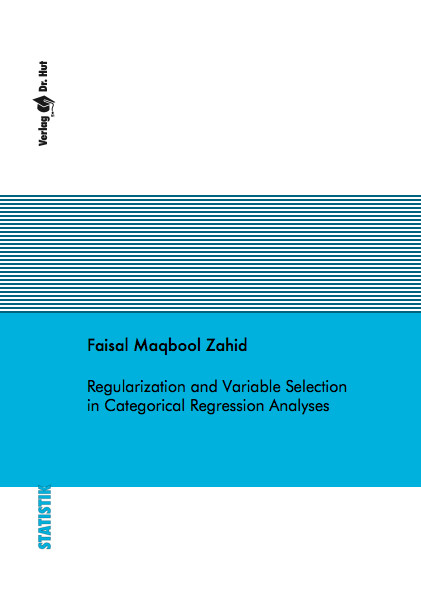Datenbestand vom 12. August 2022aktualisiert am 12. August 2022

# ISBN 978386853987560,00 € inkl. MwSt, zzgl. Versand

978-3-86853-987-5, Reihe Statistik

Faisal Maqbool Zahid
Regularization and Variable Selection in Categorical Regression Analyses

141 Seiten, Dissertation Ludwig-Maximilians-Universität München (2011), Softcover, A5

## Zusammenfassung / Abstract

In regression analyses, multivariate generalized linear models (GLM) are used for modelling the multi-categories response models. Typically, the maximum likelihood method is used for estimating the parameters. However, the use of maximum likelihood estimation (MLE) severely limits the number of predictors in multi-categories response models. The ML estimates become unstable or even do not exist with large number of parameters relative to the sample size. If sample size is less than the number of parameters or if there is complete separation in the data, the maximum likelihood approach is bound to fail. In this thesis, these problems with the usual maximum likelihood are addressed and solutions are developed for multi-categories response models. The methodology developed in the thesis can be dichotomized into two parts.

In the first instance some regularization techniques are developed that resolve the problems with MLE without performing variable selection. A response shrinkage estimation method for multinomial logit models and proportional odds models is developed that shrinks the parameter estimates without using any penalty term. Rather the observed responses are sharpened to make them close to the underlying probabilities. The resulting estimates then have smaller mean squared error and smaller bias than usual MLE and have improved existence than ML estimates. Also L2 penalty is used with symmetric side constraints for multinomial logit models. The penalized estimates in multinomial logit models are dependent on the choice of the reference category. A ridge estimation procedure is developed that uses the symmetric side constraints instead of a reference category constraint. The use of symmetric side constraints makes the ridge estimates independent of the choice of reference category. A ridge estimation procedure is also designed for ordinal response models with categorical predictors.

The second issue addressed in this thesis is variable selection in high-dimensional data structures. Variable selection is an important and critical issue in multi-categories response models. Likelihood-based boosting is used for variable selection to fit the sparse models. A boosting algorithm called multinomBoost is developed for multinomial logit models that not only fits the model but also selects the relevant predictors (factors) rather than parameters. For the proportional odds models, a boosting algorithm called pom-Boost is developed that fits the model with implicit variable selection. For both of these boosting algorithms a quadratic penalty is used to obtain the weak learners. In case of ordinal predictors the differences between the parameters of adjacent categories are penalized to avoid high jumps and as a result we get a smoother coefficient vector. Both algorithms also allow to include some covariates as mandatory in the model.